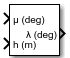Documentation

# Geodetic to Geocentric Latitude

Convert geodetic latitude to geocentric latitude

## Library

Utilities/Axes Transformations## Description

The Geodetic to Geocentric Latitude block converts a geodetic latitude (μ) into geocentric latitude (λ). Geocentric latitude at the planet surface $\left({\lambda }_{s}\right)$ is defined by flattening (f), and geodetic latitude in the following relationship.

`${\lambda }_{s}=\text{atan}\left({\left(1-f\right)}^{2}\mathrm{tan}\mu \right)$`

Geocentric latitude is defined by mean sea-level altitude (h), geodetic latitude, radius of the planet $\left({r}_{s}\right)$ and geocentric latitude at the planet surface in the following relationship.

`$\lambda =\text{atan}\left(\frac{h\mathrm{sin}\mu +{r}_{s}\mathrm{sin}{\lambda }_{s}}{h\mathrm{cos}\mu +{r}_{s}\mathrm{cos}{\lambda }_{s}}\right)$`

## Parameters

Units

Specifies the parameter and output units:

Units

Altitude

`Metric (MKS)`

Meters

Meters

`English`

Feet

Feet

This option is only available when Planet model is set to `Earth (WGS84)`.

Planet model

Specifies the planet model to use: `Custom` or ```Earth (WGS84)```.

Flattening

Specifies the flattening of the planet. This option is only available with Planet model set to `Custom`.

Specifies the radius of the planet at its equator. The units of the equatorial radius parameter should be the same as the units for altitude. This option is only available with Planet model set to `Custom`.

## Inputs and Outputs

InputDimension TypeDescription

First

ScalarContains the geodetic latitude, in degrees. Latitude values can be any value. However, values of +90 and -90 may return unexpected values because of singularity at the poles.

Second

ScalarContains the mean sea-level altitude (MSL).
OutputDimension TypeDescription

First

ScalarContains the geocentric latitude, in degrees.

## Assumptions and Limitations

This implementation generates a geocentric latitude that lies between ±90 degrees.

## Reference

Stevens, B. L., and F. L. Lewis, Aircraft Control and Simulation, John Wiley & Sons, New York, 1992.

#### Introduced before R2006a

##### SupportGet trial now